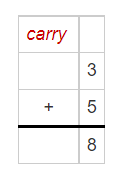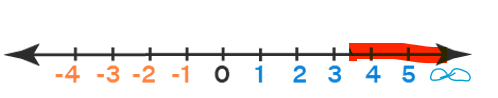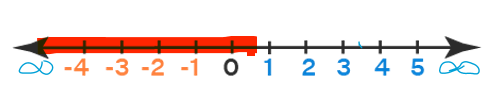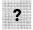# Math in Focus Grade 7 Chapter 4 Answer Key Algebraic Equations and Inequalities

Practice the problems of Math in Focus Grade 7 Workbook Answer Key Chapter 4 Algebraic Equations and Inequalities to score better marks in the exam.

## Math in Focus Grade 7 Course 2 A Chapter 4 Answer Key Algebraic Equations and Inequalities

### Math in Focus Grade 7 Chapter 4 Quick Check Answer Key

Solve each equation.

Question 1.
x + 4 = 10
6

Explanation:
x + 4 = 10
x = 10 -4

x = 6

Question 2.
x – $$\frac{1}{2}$$ = 2
2(1/2)

Explanation:
x – (1/2) = 2

x = 2 + (1/2)

x = 2 (1/2)

Question 3.
$$\frac{1}{5}$$x = 3
15

Explanation:
(1/5)x = 3
x=  3 × 5

x = 15

Question 4.
1.2x = 2.4
2

Explanation:
1.2x=2.4

x= 2.4 ÷ 1.2

x= 2

State whether each statement is True or False.

Question 5.
x = 1 gives the solution of the algebraic equation 3x + 5 = 8.
True

Explanation:
3x + 5 = 8

Given, x = 1. Substitute x= 1 in the equation.

3(1) + 5  = 8

8 = 8

Hence proved.Question 6.
y = 2 gives the solution of the algebraic equation 6y – 3 = 8.
False

Explanation:
6y – 3 = 8

Given y = 2, substitute in the equation.

6(2) – 3 = 8

12 – 3 = 8

9 ≠ 8

So False.

Question 7.
z = 6 gives the solution of the algebraic equation $$\frac{z}{3}$$ = 3.
False

Explanation:
$$\frac{z}{3}$$ = 3

(z/3) = 3

Given, z = 6, Substitute it in the equation.

(6/3) = 3

2 ≠ 3

So  false.

Question 8.
w = 3 gives the solution of the algebraic equation 2w = 6.
True

Explanation:
Given equation, 2w = 6.

Given w=3, substitute it in the equation.

2(3) = 6

6 = 6

So the equation is true.

Draw a number line to represent each inequality.

Question 9.
x ≥ 3.5Explanation:
Given equation, x ≥ 3.5

In the equation , x can be any number which is equal to (or) more than 3.5.

It is represented in the number line as given above.

Question 10.
y < $$\frac{1}{2}$$Explanation:
Given equation, y < (1/2)

In the equation, y is a number which is less than (1/2)

It is represented in the number line as given above.

Complete eachwith =, >, or <.

Question 11.
1112
11 < 12

Question 12.
-9-7
-9  <  -7

Question 13.
25 ∙ (-1)(-1) ∙ 25
25 ∙ (-1)  =  (-1) ∙ 25

Question 14.
3 ÷ (-1)(-1) ÷ 3
3 ÷ (-1) = (-1) ÷ 3

Use x to represent the unknown quantity. Write an algebraic inequality for each statement.

Question 15.
The box can hold less than 70 pounds.
x < 70

Explanation:
Let us consider the box be “x”.

As the box can hold less than 70 pounds.

Hence the equation x < 70 .

Question 16.
You have to be at least 17 years old to qualify for the contest.
x ≥ 17

Explanation:
“At least” means it should be minimum of 17 or more than that.

Given, you have to be at least 17 years old to qualify for the contest.

Hence the equation, x ≥ 17.

Question 17.
The width of luggage that you can carry onto the plane is at most 17 inches.
y ≤ 17

Explanation:
“At most” means it should be maximum of 17 nor less than that, but not more than 17.

As, The width of luggage that you can carry onto the plane is at most 17 inches.

Hence the equation, y ≤ 17

Question 18.
There are more than 120 people standing in line for the roller coaster.